# E-PolyLearning

 31. The hydraulic radius for flow in a rectangular duct of cross-sectional dimension H, W is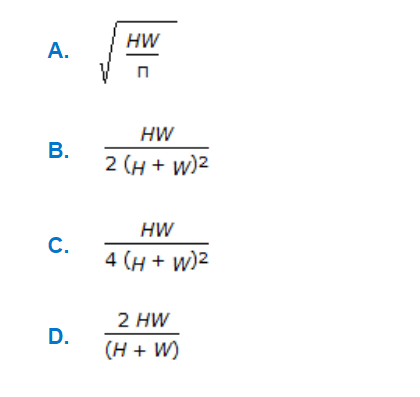a. A b. B c. C d. D

 32. A pipe has a porous section of length L as shown in the figure. Velocity at the start of this section of V₀. If fluid leaks into the pipe through the porous section at a volumetric rate per unit area q(x/L)², what will be axial velocity in the pipe at any x ? Assume incompressible one dimensional flow i.e., no gradients in the radial direction.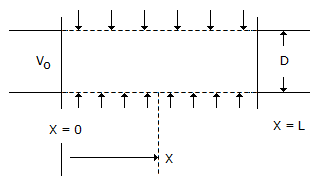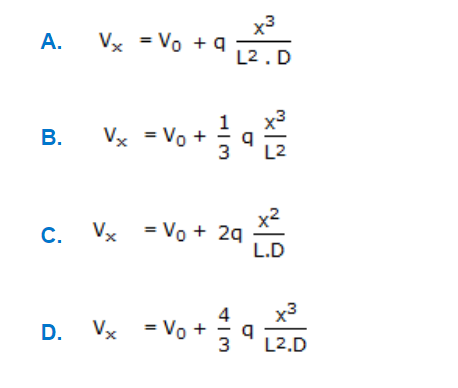a. A b. B c. C d. D
 33. A free jet of water of cross-sectional area 0.01 m² and a velocity of 20 m/s strikes a plate and then flows in a plane parallel to the plate as shown in the figure below. The horizontal component of the force on the support is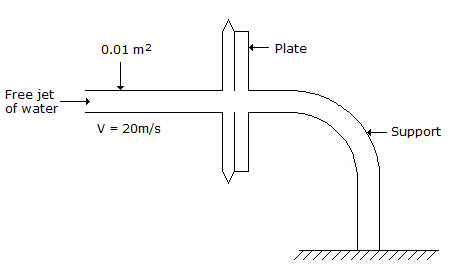a. 200 N b. 400 N c. 2000 N d. 4000 N
 34. Pressure drop for laminar fluid flow through a circular pipe is given by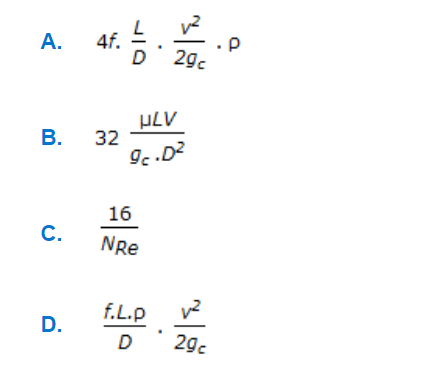a. A b. B c. C d. D
 35. Drag co-efficient for motion of spherical particles in a stationary fluid in the stoke's law range is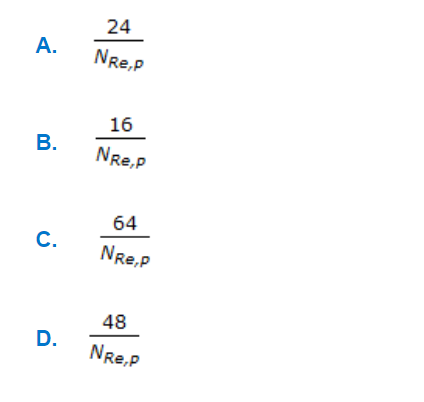a. A b. B c. C d. D